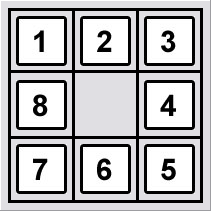# Solving 8-puzzle problem using A algorithm

suggest change
Problem definition:

An 8 puzzle is a simple game consisting of a 3 x 3 grid (containing 9 squares). One of the squares is empty. The object is to move to squares around into different positions and having the numbers displayed in the “goal state”.Given an initial state of 8-puzzle game and a final state of to be reached, find the most cost-effective path to reach the final state from initial state.

Initial state:
```_ 1 3
4 2 5
7 8 6```
Final state:
```1 2 3
4 5 6
7 8 _```
Heuristic to be assumed:

Let us consider the Manhattan distance between the current and final state as the heuristic for this problem statement.

```h(n) = | x - p | + | y - q |
where x and y are cell co-ordinates in the current state
p and q are cell co-ordinates in the final state```
Total cost function:

So the total cost function `f(n)` is given by,

`f(n) = g(n) + h(n), where g(n) is the cost required to reach the current state from given initial state`
Solution to example problem:

First we find the heuristic value required to reach the final state from initial state. The cost function, g(n) = 0, as we are in the initial state

`h(n) = 8`

The above value is obtained, as `1` in the current state is 1 horizontal distance away than the `1` in final state. Same goes for `2`, `5`, `6`. `\_` is 2 horizontal distance away and 2 vertical distance away. So total value for `h(n)` is 1 + 1 + 1 + 1 + 2 + 2 = 8. Total cost function `f(n)` is equal to 8 + 0 = 8.

Now, the possible states that can be reached from initial state are found and it happens that we can either move `\_` to right or downwards.

So states obtained after moving those moves are:

```1 _ 3    4 1 3
4 2 5    _ 2 5
7 8 6    7 8 6
(1)      (2)```

Again the total cost function is computed for these states using the method described above and it turns out to be 6 and 7 respectively. We chose the state with minimum cost which is state (1). The next possible moves can be Left, Right or Down. We won’t move Left as we were previously in that state. So, we can move Right or Down.

Again we find the states obtained from (1).

```1 3 _    1 2 3
4 2 5    4 _ 5
7 8 6    7 8 6
(3)      (4)```
1. leads to cost function equal to 6 and (4) leads to 4. Also, we will consider (2) obtained before which has cost function equal to 7. Choosing minimum from them leads to (4). Next possible moves can be Left or Right or Down. We get states:
```1 2 3    1 2 3    1 2 3
_ 4 5    4 5 _    4 8 5
7 8 6    7 8 6    7 _ 6
(5)      (6)      (7)```

We get costs equal to 5, 2 and 4 for (5), (6) and (7) respectively. Also, we have previous states (3) and (2) with 6 and 7 respectively. We chose minimum cost state which is (6). Next possible moves are Up, and Down and clearly Down will lead us to final state leading to heuristic function value equal to 0.